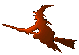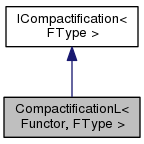Hex  1.0 Hydrogen-electron collision solver
CompactificationL< Functor, FType > Class Template Reference

Compactification of a function from (-∞,b] to (-1,1]. More...

#include <compact.h>

Inheritance diagram for CompactificationL< Functor, FType >:[legend]
Collaboration diagram for CompactificationL< Functor, FType >:[legend]

## Public Member Functions

CompactificationL (Functor f, double b=0., bool limit=true, double L=1.0)

double scale (double x) const
Scale value from the original interval [a,b] into compactified interval [-1,1]. More...

double unscale (double t) const
Unscale value from the compactified interval [-1,1] into the original interval [a,b]. More...

double Jacobian (double t) const
Evaluate Jacobian of the transformation. More...

FType operator() (double t) constPublic Member Functions inherited from ICompactification< FType >
virtual ~ICompactification ()

## Detailed Description

### template<class Functor, typename FType> class CompactificationL< Functor, FType >

Transform arbitrary function so that its definition range will be $$t \in [-1,1]$$, for original interval $$x \in [a,b]$$. The following formulas are used:

$x = b - L \frac{1+t}{1-t} \Leftrightarrow t = \frac{x - b + L}{x - b + L} \ .$

Note that in this case the monotonic character is reverse.

## Constructor & Destructor Documentation

template<class Functor , typename FType >
 CompactificationL< Functor, FType >::CompactificationL ( Functor f, double b = 0., bool limit = true, double L = 1.0 )
inline

Constructor of the class The parameter "b" specifies original definition interval (-∞,b].

## Member Function Documentation

template<class Functor , typename FType >
 double CompactificationL< Functor, FType >::Jacobian ( double t ) const
inlinevirtual
template<class Functor , typename FType >
 FType CompactificationL< Functor, FType >::operator() ( double t ) const
inlinevirtual

Evaluate the compactified function.

Parameters
 t Value from the compactified interval [-1,1].

Implements ICompactification< FType >.

template<class Functor , typename FType >
 double CompactificationL< Functor, FType >::scale ( double x ) const
inlinevirtual
template<class Functor , typename FType >
 double CompactificationL< Functor, FType >::unscale ( double t ) const
inlinevirtual

The documentation for this class was generated from the following file: